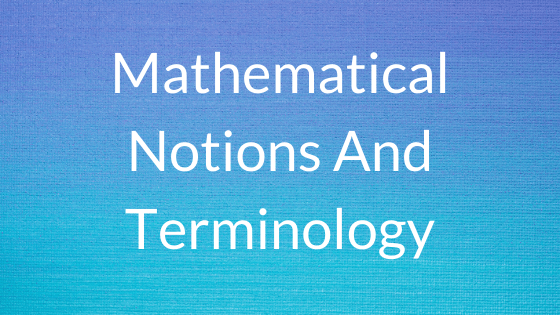# Mathematical Notions And Terminology## Mathematical Notions And Terminology

As in any mathematical subject , we begin with a discussion of the basic mathematical objects, tools, and notation that we expect to use.

## SET

A set is a group of objects represented as a unit. Sets may contain any type of object, including numbers, symbols, and even other sets.

• The objects in a set are called its elements or members.
• For sets, we use a type of picture called a Venn diagram
• The set with 0 members is called the empty set

## SEQUENCES AND TUPLES

A sequence of objects is a list of these objects in some order. As with sets, sequences may be finite or infinite. Finite sequences often are called tuples

• We usually designate a sequence by writing the list within parentheses.
• In a set, the order doesn’t matter, but in a sequence it does
• Repetition does matter in a sequence, but it does not matter in a set

## FUNCTIONS AND RELATIONS

A function is an object that sets up input-output relationship. A function takes an input and produces an output.

• A function is called a mapping if f(a) = b, we say that f maps a to b.
• The set of possible inputs to the function is called its domain.
• The outputs of a function come from a set called its range.
• The notation for saying that f is a function with domain D and range R is f : D –> R
• A function may not necessarily use all the elements of the specified range.
• A function that does use all the elements of the range is said to be onto the range.

## GRAPHS

An undirected graph, or simply a graph, is a set of points with lines connecting some of the points.

• The points are called nodes or vertices, and the lines are called edges.
• The number of edges at a particular node is the degree of that node.
• Graphs frequently are used to represent data.
• A path in a graph is a sequence of nodes connected by edges.
• A simple path is a path that doesn’t repeat any nodes.
• A graph is connected if every two nodes have a path between them.
• A path is a cycle if it starts and ends in the same node.
• A simple cycle is one that contains at least three nodes and repeats only the first and last nodes.
• A graph is a tree if it is connected and hs no simple cycles